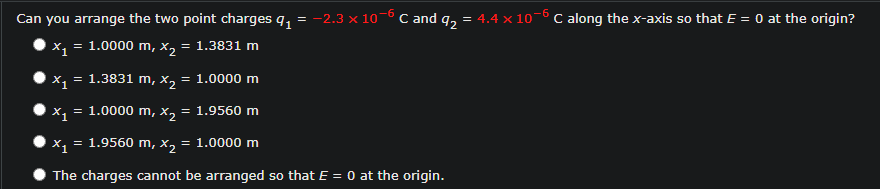# Question Solved1 AnswerCan you arrange the two point charges 9, = -2.3 x 10-6C and q2 = 4.4 x 10-6C along the x-axis so that E = 0 at the origin? X = 1.0000 m, x2 = 1.3831 m X1 = 1.3831 m, x2 = 1.0000 m X1 = 1.0000 m, x2 = 1.9560 m X1 1.9560 m, x2 = 1.0000 m The charges cannot be arranged so that E = 0 at the origin.Transcribed Image Text: Can you arrange the two point charges 9, = -2.3 x 10-6C and q2 = 4.4 x 10-6C along the x-axis so that E = 0 at the origin? X = 1.0000 m, x2 = 1.3831 m X1 = 1.3831 m, x2 = 1.0000 m X1 = 1.0000 m, x2 = 1.9560 m X1 1.9560 m, x2 = 1.0000 m The charges cannot be arranged so that E = 0 at the origin.
More
Transcribed Image Text: Can you arrange the two point charges 9, = -2.3 x 10-6C and q2 = 4.4 x 10-6C along the x-axis so that E = 0 at the origin? X = 1.0000 m, x2 = 1.3831 m X1 = 1.3831 m, x2 = 1.0000 m X1 = 1.0000 m, x2 = 1.9560 m X1 1.9560 m, x2 = 1.0000 m The charges cannot be arranged so that E = 0 at the origin.